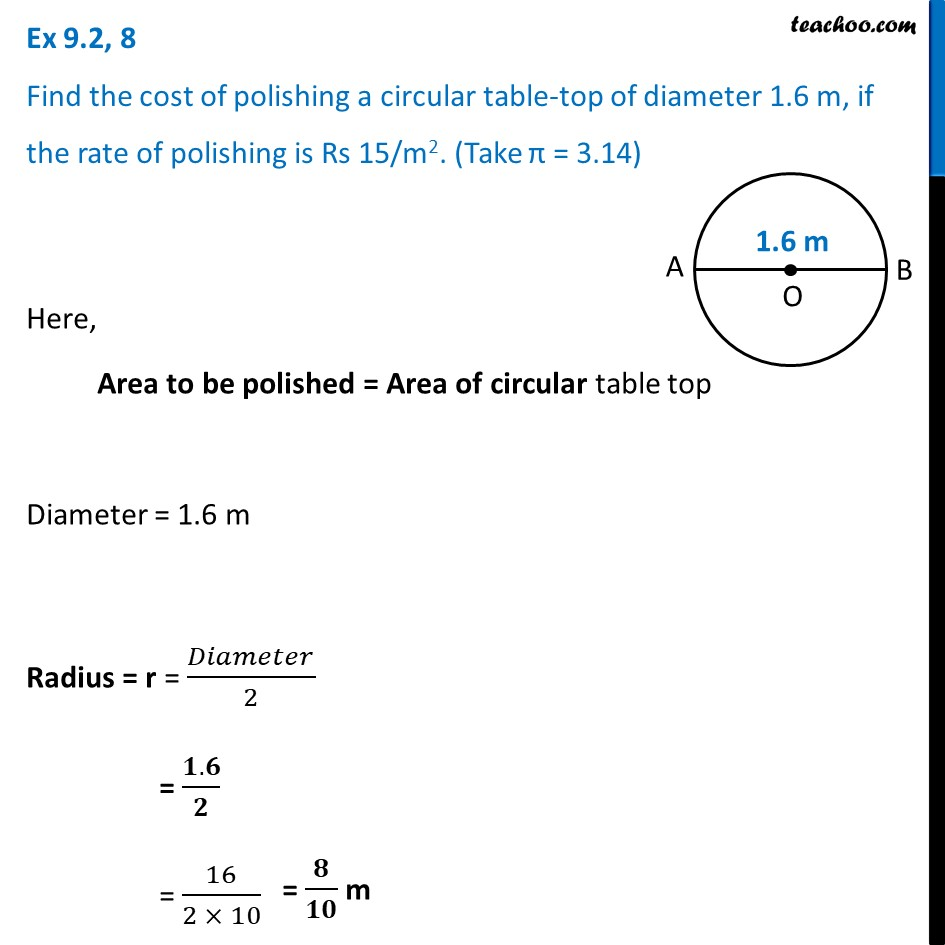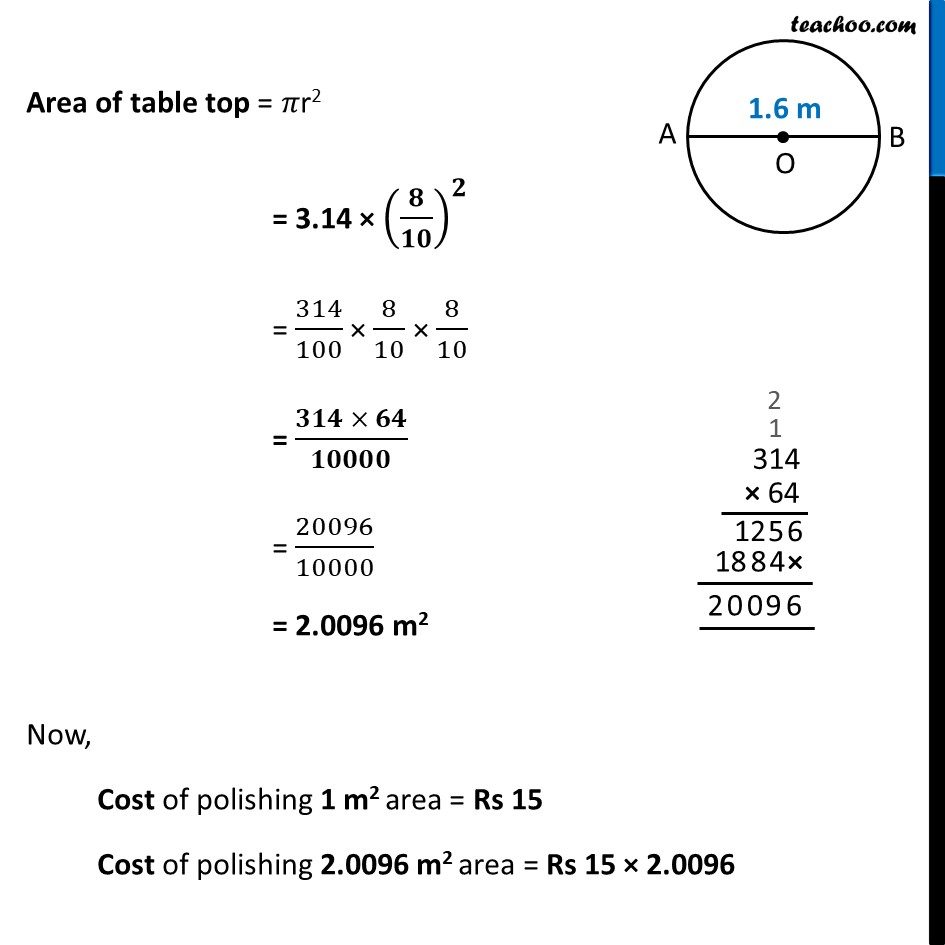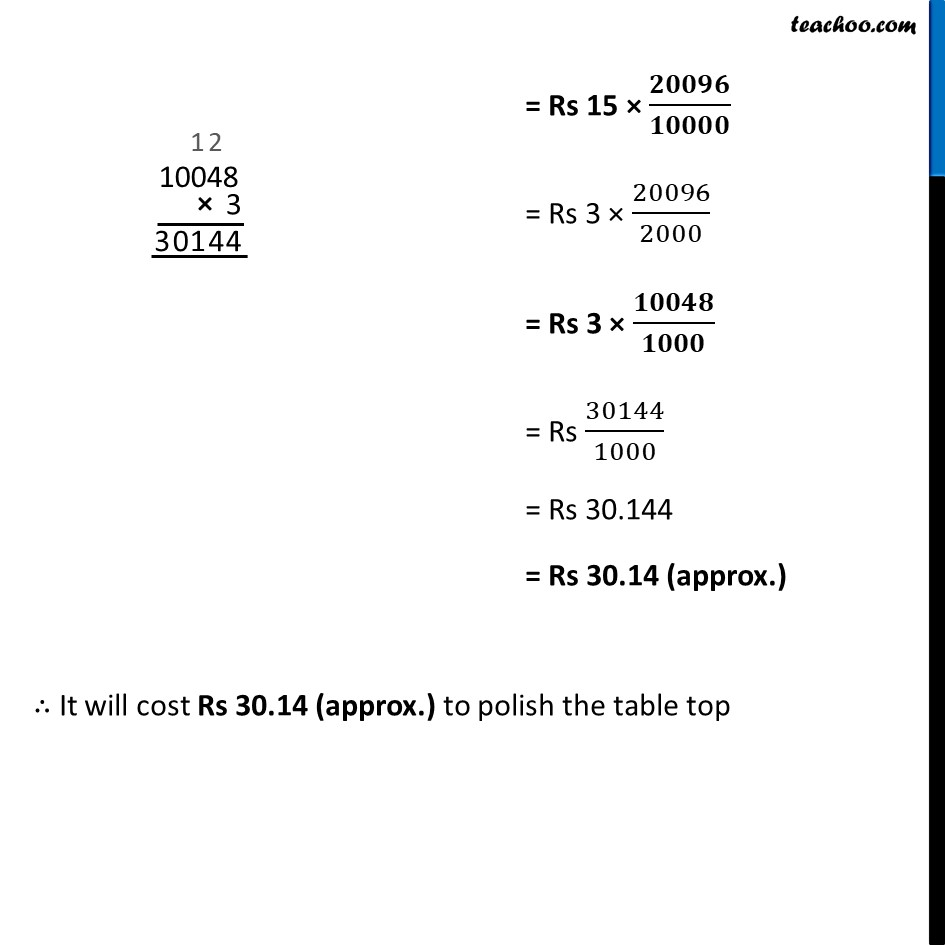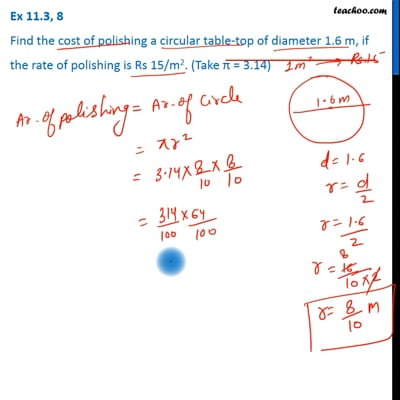Ex 9.2

Chapter 9 Class 7 Perimeter and Area
Serial order wiseThis video is only available for Teachoo black users

Learn in your speed, with individual attention - Teachoo Maths 1-on-1 Class

### Transcript

Ex 9.2, 8 Find the cost of polishing a circular table-top of diameter 1.6 m, if the rate of polishing is Rs 15/m2. (Take π = 3.14) Here, Area to be polished = Area of circular table top Diameter = 1.6 m Radius = r = 𝐷𝑖𝑎𝑚𝑒𝑡𝑒𝑟/2 = (𝟏.𝟔)/𝟐 = 16/(2 × 10) Area of table top = 𝜋r2 = 3.14 × (𝟖/𝟏𝟎)^𝟐 = 314/100 × 8/10 × 8/10 = (𝟑𝟏𝟒 × 𝟔𝟒)/𝟏𝟎𝟎𝟎𝟎 = 20096/10000 = 2.0096 m2 Now, Cost of polishing 1 m2 area = Rs 15 Cost of polishing 2.0096 m2 area = Rs 15 × 2.0096 = Rs 15 × 𝟐𝟎𝟎𝟗𝟔/𝟏𝟎𝟎𝟎𝟎 = Rs 3 × 20096/2000 = Rs 3 × 𝟏𝟎𝟎𝟒𝟖/𝟏𝟎𝟎𝟎 = Rs 30144/1000 = Rs 30.144 = Rs 30.14 (approx.) ∴ It will cost Rs 30.14 (approx.) to polish the table top